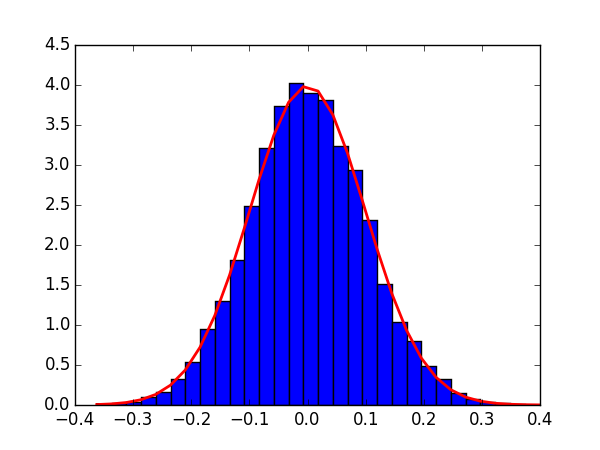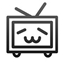• np random normal 到正态分布的拟合


先看伟大的高斯分布（Gaussian Distribution）的概率密度函数（probability density function）：

f(x)=12π − −  √ σ exp(−(x−μ) 2 2σ 2  ) f(x)=12πσexp⁡(−(x−μ)22σ2)
），对应于
np.random.normal(loc=0, scale=1, size)。

采样（sampling）
# 从某一分布（由均值和标准差标识）中获得样本mu, sigma = 0, .1s = np.random.normal(loc=mu, scale=sigma, size=1000)
123
也可使用scipy库中的相关api（这里的类与函数更符合数理统计中的直觉）：
import scipy.stats as stmu, sigma = 0, .1s = st.norm(mu, sigma).rvs(1000)
123
校验均值和方差：
>>> abs(mu < np.mean(s)) < .01True>>> abs(sigma-np.std(s, ddof=1)) < .01True            # ddof，delta degrees of freedom，表示自由度            # 一般取1，表示无偏估计，      
123456
拟合
我们看使用matplotlib.pyplot便捷而强大的语法如何进行高斯分布的拟合：
import matplotlib.pyplot as pltcount, bins, _ = plt.hist(s, 30, normed=True)        # normed是进行拟合的关键        # count统计某一bin出现的次数，在Normed为True时，可能其值会略有不同plt.plot(bins, 1./(np.sqrt(2*np.pi)*sigma)*np.exp(-(bins-mu)**2/(2*sigma**2), lw=2, c='r')plt.show()
123456
或者：
s_fit = np.linspace(s.min(), s.max())plt.plot(s_fit, st.norm(mu, sigma).pdf(s_fit), lw=2, c='r')
12再分享一下我老师大神的人工智能教程吧。零基础！通俗易懂！风趣幽默！还带黄段子！希望你也加入到我们人工智能的队伍中来！https://blog.csdn.net/jiangjunshow
展开全文• 在使用numpy的时候，我们经常会使用到np.random一系列的有关函数,来创建ndarray 数组。random代表随机的意思，指ndarray中的数是随机数。后面的函数表示随机生成的ndarray需要符合什么样的条件。因为其太多，所以...
• np.random.rand用法 觉得有用的话,欢迎一起讨论相互学习~...np.random.rand(a1,a2,a3…)生成形状为(a1,a2,a3…),[0,1)之间的均匀分布随机数 np.random.rand(3,2) # array([[ 0.14022471, 0.96360618], #random...
np.random.rand用法
觉得有用的话,欢迎一起讨论相互学习~生成特定形状下[0,1)下的均匀分布随机数
np.random.rand(a1,a2,a3…)生成形状为(a1,a2,a3…),[0,1)之间的 均匀分布 随机数
np.random.rand(3,2)
array([[ 0.14022471,  0.96360618],  #random
[ 0.37601032,  0.25528411],  #random
[ 0.49313049,  0.94909878]]) #random


np.random.randn用法
生成特定形状下的正态分布随机数
正态分布即高斯分布 np.random.randn(a1,a2,a3…)生成形状为(a1,a2,a3…)的 均匀分布 随机数如果想要生成满足

N

(

μ

,

σ

2

)

N(\mu, \sigma^2)

其中

μ

表

示

平

均

值

,

σ

2

表

示

方

差

\mu表示平均值, \sigma^2表示方差

, 可以使用语句sigma * np.random.randn(...) + mu
>>> np.random.randn()
2.1923875335537315 #random

Two-by-four array of samples from N(3, 6.25):

>>> 2.5 * np.random.randn(2, 4) + 3
array([[-4.49401501,  4.00950034, -1.81814867,  7.29718677],  #random
[ 0.39924804,  4.68456316,  4.99394529,  4.84057254]]) #random

展开全文numpy
• 0. np.random.RandomState 计算机实现的随机数生成通常为伪随机数生成器，为了使得具备随机性的代码最终的结果可复现，需要设置相同的种子值； np.random.randn(…) ⇒  rng = np.random.RandomState(123)...
 0. np.random.RandomState
计算机实现的随机数生成通常为伪随机数生成器，为了使得具备随机性的代码最终的结果可复现，需要设置相同的种子值；
np.random.randn(…) ⇒
rng = np.random.RandomState(123)rng.randn(…)
1. np.random.uniform()
首先从numpy.random.uniform说起（也即其他函数是对该函数的进一步封装）。
numpy.random.uniform(low=0.0, high=1.0, size=None)
1
1
顾名思义，从一个均匀分布（[low, high)：半开区间）中进行采样。
例如产生[1, 2)（五行五列）：
>>> import numpy
>>> np.random.uniform(1, 2, (5, 5))
array([[ 1.16902081,  1.90805984,  1.30759311,  1.90598113,  1.32047656],
[ 1.58571077,  1.88009484,  1.66531622,  1.0262826 ,  1.40534658],
[ 1.81087389,  1.87981194,  1.65670468,  1.46972606,  1.66454007],
[ 1.81041299,  1.52561204,  1.79701198,  1.17840313,  1.86364978],
[ 1.72654371,  1.92870279,  1.11207754,  1.5091156 ,  1.35108628]])
1234567
1234567
2. np.random.random is Alias for np.random.random_sample
alias: 别名；
>>> id(np.random.random) == id(np.random.random_sample)
True
12
12
numpy.random.random(size=None)
# 已指定区间为[0., 1.)，自然是float类型
12
12
必须以元组形式指定size：
>>> np.random.random((2, 3))
array([[ 0.14367   ,  0.48649543,  0.38761876],
[ 0.11565701,  0.6474381 ,  0.84394864]])
>>> np.random.random(2, 3)
TypeError: random_sample() takes at most 1 positional argument (2 given)
12345
12345
3. np.random.rand: a convenience function for np.random.uniform(0, 1)
numpy.random.rand(d0, d1, ..., dn)
# 以参数列表的形式指定参数，而非元组
# 内部指定区间为[0., 1.)
123
123
>>> np.random.rand(2, 2)
array([[ 0.9978749 ,  0.43597209],
[ 0.30804578,  0.9632462 ]])

>>> np.random.rand((2, 2))
TypeError: an integer is required
123456123456
4. 使用 np.random.RandomState() 获取随机数生成器
>> rng = np.random.RandomState(22)
>> rng.rand(2, 3)
array([[ 0.48168106,  0.42053804,  0.859182  ],
[ 0.17116155,  0.33886396,  0.27053283]])
展开全文• numpy.random.uniform(low=0.0, high=1.0, size=None)顾名思义，从一个均匀分布（[low, high)：半开区间）中进行采样。例如产生[1, 2)（五行五列）：>>> import numpy >>> np.random.uniform(1, 2, (5, 5))
0. np.random.RandomState
计算机实现的随机数生成通常为伪随机数生成器，为了使得具备随机性的代码最终的结果可复现，需要设置相同的种子值；
np.random.randn(…) ⇒
rng = np.random.RandomState(123)rng.randn(…)
1. np.random.uniform()
首先从numpy.random.uniform说起（也即其他函数是对该函数的进一步封装）。
numpy.random.uniform(low=0.0, high=1.0, size=None)
顾名思义，从一个均匀分布（[low, high)：半开区间）中进行采样。
例如产生[1, 2)（五行五列）：
>>> import numpy
>>> np.random.uniform(1, 2, (5, 5))
array([[ 1.16902081,  1.90805984,  1.30759311,  1.90598113,  1.32047656],
[ 1.58571077,  1.88009484,  1.66531622,  1.0262826 ,  1.40534658],
[ 1.81087389,  1.87981194,  1.65670468,  1.46972606,  1.66454007],
[ 1.81041299,  1.52561204,  1.79701198,  1.17840313,  1.86364978],
[ 1.72654371,  1.92870279,  1.11207754,  1.5091156 ,  1.35108628]])
2. np.random.random is Alias for np.random.random_sample
alias: 别名；
>>> id(np.random.random) == id(np.random.random_sample)
True
numpy.random.random(size=None)
# 已指定区间为[0., 1.)，自然是float类型
必须以元组形式指定size：
>>> np.random.random((2, 3))
array([[ 0.14367   ,  0.48649543,  0.38761876],
[ 0.11565701,  0.6474381 ,  0.84394864]])
>>> np.random.random(2, 3)
TypeError: random_sample() takes at most 1 positional argument (2 given)
3. np.random.rand: a convenience function for np.random.uniform(0, 1)
numpy.random.rand(d0, d1, ..., dn)
# 以参数列表的形式指定参数，而非元组
# 内部指定区间为[0., 1.)
>>> np.random.rand(2, 2)
array([[ 0.9978749 ,  0.43597209],
[ 0.30804578,  0.9632462 ]])

>>> np.random.rand((2, 2))
TypeError: an integer is required
4. 使用 np.random.RandomState() 获取随机数生成器
>> rng = np.random.RandomState(22)
>> rng.rand(2, 3)
array([[ 0.48168106,  0.42053804,  0.859182  ],
[ 0.17116155,  0.33886396,  0.27053283]])

 numpy.random.uniform
展开全文• 1、np.random.rand()函数 语法： np.random.rand(d0,d1,d2……dn) 注：使用方法与np.random.randn()函数相同 作用： 通过本函数可以返回一个或一组服从“0~1”均匀分布的随机样本值。随机样本取值范围是[0,1)，不...python
• np.random.normal()正态分布 numpy中高斯分布的概率密度函数 numpy.random.normal(loc=0.0, scale=1.0, size=None) 参数的意义为： loc:float 概率分布的均值，对应着整个分布的中心center scale:float 概率...python
• Create an array of the given shape and populate it with random samples from a uniform distribution (均匀分布) over [0, 1). Example:>>> np.random.rand(3,2) 　array([[ 0.14022471,...
• 在机器学习和神经网络中，常常会利用Numpy库中的随机函数来生产随机数，比如随机初始化神经网络中的参数权重W... 在Numpy库中，常用使用np.random.rand()、np.random.randn()和np.random.randint()随机函数。这几个...
• np.random.randn(d0, d1, …, dn) 通过本函数可以返回一个或一组服从“0~1”均匀分布的随机样本值。随机样本取值范围是[0,1)，不包括1。 标准正态分布是以 0 为均数、以 1 为标准差的正态分布，记为 N（0，1） 1...
• np.random.rand()：生成一个[0,1)区间均匀分布的随机数，示例： np.random.rand() Out: 0.7309286434720168 np.random.rand(m, n)：生成m*n阶矩阵，矩阵内数值为[0,1)区间均匀分布的随机数，示例： np.random...python numpy 机器学习
• np.random.randn(size = ()) # 生成一个符合标准正态分布的数组，与np.random.normal(0,1,size = ()）等价 np.random.randint() # 生成固定范围内的，固定形状的数组 numpy.random.normal(loc=0.0, scale=1.0, size...python
• numpy.random.rand(d0, d1, …, dn)的随机样本位于[0, 1)中：本函数可以返回一个或一组服从**“0~1”均匀分布**的随机样本值。 numpy.random.randn(d0, d1, …, dn)是从标准正态分布中返回一个或多个样本值。  1. ...
• 目录1、numpy.random.uniform()2、np.random.randn()3、np.random.binomial() 1、numpy.random.uniform() numpy.random.uniform(low,high,size) 从一个均匀分布[low,high)中随机采样，注意定义域是左闭右开，即包含...
• np.random.rand()与np.random.randn()函数用法相同 np.random.rand()函数 作用： 返回一个或一组服从“0~1”均匀分布的随机样本值。随机样本取值范围是[0,1)，不包括1。 import numpy as np print(np.random..python
• np.random.randn()函数 语法： np.random.randn(d0,d1,d2……dn) 1. 当函数括号内没有参数时，则返回一个浮点数； 2. 当函数括号内有一个参数时，则返回秩为1的数组，不能表示向量和矩阵； 3. 当函数括号内有两个及...python
• 看了很多博客，感觉很多人对np.random.binomial()的解释都写得不是很清楚，或者写错了，或者写得很模糊费解。特别是对该函数的参数解释非常的模糊、不清楚。本文以二项式分布的理解为起点，对该函数进行解释，欢迎...binomial
• data = np.random.normal(size=100) print(data) 打印： 直方图： plt.hist(data) 例： """normal：正态分布平均值9000标准差3000 300个数值""" data=np.random.normal(9000,3000,si...python numpy
• nprandom模块用于生成随机数，常用函数的用法： numpy.random.rand(d0, d1, …, dn)：生成一个[0,1)之间的随机浮点数或N维浮点数组。 #numpy.random.rand(d0, d1, ..., dn) import numpy as np #无参 np.random....
• np.random.randn及np.random.rand的区别 numpy中有一些常用的用来产生随机数的函数，randn()和rand()就属于这其中。 numpy.random.randn(d0, d1, …, dn)是从标准正态分布中返回一个或多个样本值。 numpy.random....python numpy
• np.random.rand()函数 语法： np.random.rand(d0,d1,d2……dn) 注：使用方法与np.random.randn()函数相同 作用： 通过本函数可以返回一个或一组服从“0~1”均匀分布的随机样本值。随机样本取值范围是[0,1)，不包括...numpy python
• 在最近的学习中遇到了这两个函数，详细说一下这两个函数的使用方法： ...更清晰的说，即当你把设置为seed(0)，则你每次运行代码第一次用np.random.rand()产生的随机数永远是0.5488135039273248；第二次用n...numpy Python...